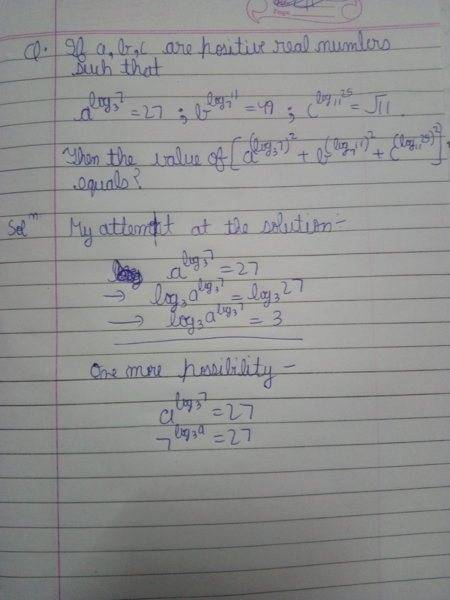# Logarithm function

## Homework Statement

I have attached image of question.[/B]

## Homework Equations

all the properties of log
a^(logₘn)=n^(logₘa)[/B]

## The Attempt at a Solution

in the attached image[/B]

#### Attachments

George Jones
Staff Emeritus
Gold Member
##\log _3 \left( a^{\log _3 7} \right)## is of the form ##\log _3 \left( a^y \right)##, Can you simplify this further?

##\log _3 \left( a^{\log _3 7} \right)## is of the form ##\log _3 \left( a^y \right)##, Can you simplify this further?
and then

jedishrfu
Mentor
and then
You tell us, George gave you a hint.

You tell us, George gave you a hint.
OK by this method I got a=20 b=38 c=√(11)-25
Then they are in power of log₃7,log₇11 and log₁₁25.How to solve these powers

SammyS
Staff Emeritus
Homework Helper
Gold Member
OK by this method I got a=20 b=38 c=√(11)-25
Then they are in power of log₃7,log₇11 and log₁₁25.How to solve these powers
The problem states that a, b, and c are positive. The value you give for c, ##\ \sqrt{11\,}-25\,,\ ## is negative.

Moreover, as it turns out, a, b, and c are all irrational. Fortunately, you do not need to solve for any of them to solve this problem.

Here is a hint that's different than the one given by George Jones.
Notice that ##\displaystyle \ X^{Y^ 2}=X^{Y\cdot Y}=\left(X^Y\right)^Y \ ##​
.